TRIGONOMETRIC FORMULAS

INTRODUCTION

Trigonometry is the study or measurement of triangles.   Central to this endeavor are the trigonometric formulas for the sine and cosine of the sum of two angles which can be used to derive all the other trigonometry formulas for some twenty in total.

SINE AND COSINE OF THE SUM OF TWO ANGLES

To start, we first draw two right triangles,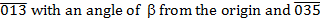and, each having one angle, α or β respectively, and then stack them one on the other as shown below.   We then draw the lines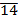and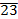to create the right triangle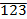which also contains the angle α.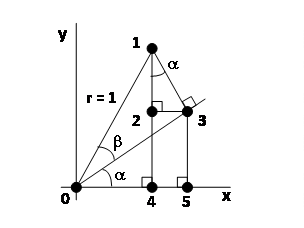Recalling the definition of the sides of a right triangle, as follows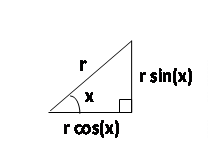The vertical height of the triangle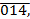in the first figure is as follows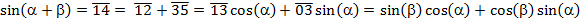But considering the triangles,, andwe can also write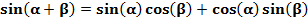And so rearranging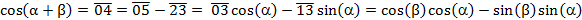The horizontal width of triangleis given asAnd from the triangles,, andwe can write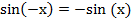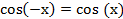And so rearranging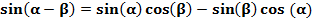SINE AND COSINE OF THE DIFFERENCE OF TWO ANGLES

From simple geometric considerations we can write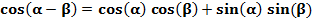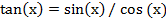These allow us to write the formulas for the difference between two angles as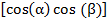TANGENT OF THE SUM AND DIFFERENCE OF TWO ANGLES

For the tangent, we can write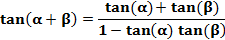so that we can write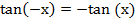by dividing the top and bottom by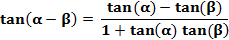, we getAnd likewise by noting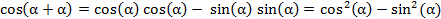we get the result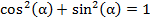DOUGLE ANGLE FORMULAS

If we set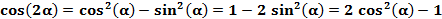then we can write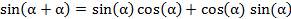But from the Pythagorean Theorem we can also write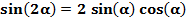so that we can write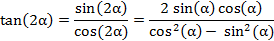In like manner we can write the double angle sine formula as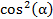or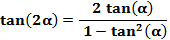And for the tangent we can writeor by dividing top and bottom bywe can write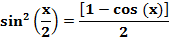HALF ANGLE FORMULAS

If we set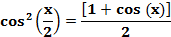, then by recalling the relationship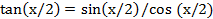we can write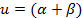And from the relationshipwe can write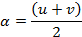TRIGONOMETRIC SUMS AS PRODUCTS

If we set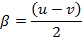and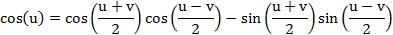, then we can rewrite our original angles as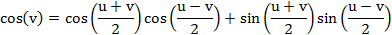and substituting these into the formulas for the cosine of the sum and difference of two angles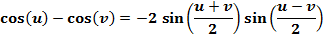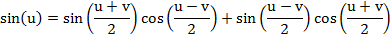And by adding and subtracting these two equations, we have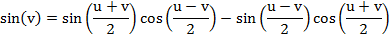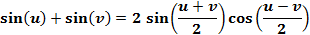And using the formulas for the sine of the sum and difference of two angles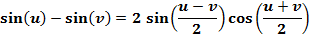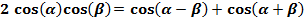And by adding and subtracting these two equations, we have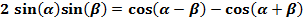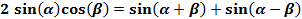TRIGONOMETRIC PRODUCTS AS SUMS

We can return to the original angles and rearrange the trigonometric sums as products on the left hand side.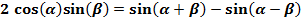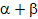FINAL NOTE

The discerning student will note an implicit assumption in that the sum of two angles is assumed to be less than 90 degrees.   While strictly correct, we can nevertheless extend the formulas for the sine and cosine of two angles, when that sum places the angle in the other three quadrants, without change.  From that point no further such assumptions need be made.